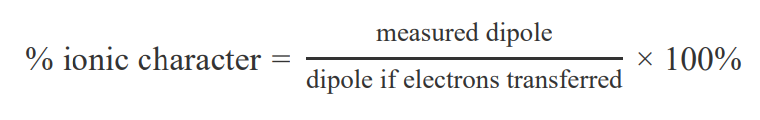# Problem: Describe the molecular dipole of OCl2.The molecular dipole is:A) zero.B) measurable and directed toward chlorine.C) measurable and directed toward oxygen.Molecules can be polar because of the unsymmetrical distribution of electrons. The dipole moment, μmu, is a measure of the net polarity and is defined as the charge QQ, in coulombs, times the distance between the charges, rr, in meters:μ = Q × rThe SI unit of dipole moment is the coulomb-meter (C ⋅ m), but another common unit is the debye (D). The two are related as3.336 × 10−30 C ⋅ m = 1 DThe percent ionic character is a comparison of the measured dipole moment of the bond to the expected dipole moment if electrons are instead transferred:The dipole moment if electrons are completely transferred is one for which a full unit of charge (1.60 × 10 − 19 C) exists on each end of the bond.

###### FREE Expert Solution
100% (100 ratings)View Complete Written Solution
###### Problem Details

Describe the molecular dipole of OCl2.

The molecular dipole is:

A) zero.
B) measurable and directed toward chlorine.
C) measurable and directed toward oxygen.

Molecules can be polar because of the unsymmetrical distribution of electrons. The dipole moment, μmu, is a measure of the net polarity and is defined as the charge QQ, in coulombs, times the distance between the charges, rr, in meters:

μ = Q × r

The SI unit of dipole moment is the coulomb-meter (C ⋅ m), but another common unit is the debye (D). The two are related as

3.336 × 10−30 C ⋅ m = 1 D

The percent ionic character is a comparison of the measured dipole moment of the bond to the expected dipole moment if electrons are instead transferred:The dipole moment if electrons are completely transferred is one for which a full unit of charge (1.60 × 10 − 19 C) exists on each end of the bond.